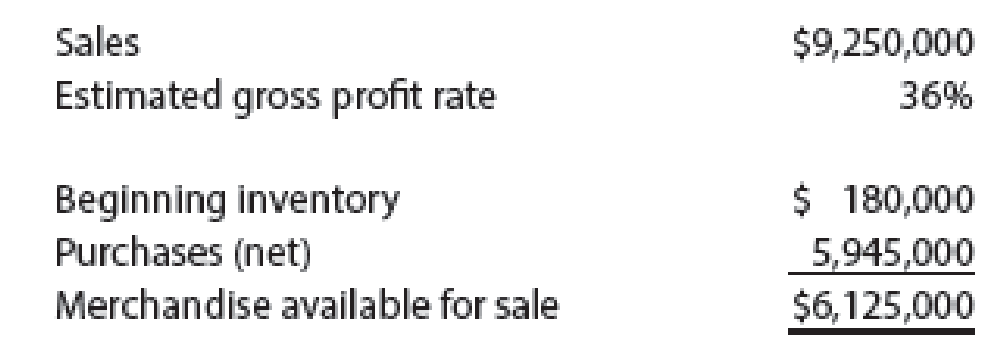# Gross profit method Based on the following data, estimate the cost of the ending inventory:### Financial And Managerial Accounting

15th Edition
WARREN + 1 other
Publisher: Cengage Learning,
ISBN: 9781337902663

#### Solutions

Chapter
Section### Financial And Managerial Accounting

15th Edition
WARREN + 1 other
Publisher: Cengage Learning,
ISBN: 9781337902663
Chapter 6, Problem 25E
Textbook Problem
1 views

## Gross profit methodBased on the following data, estimate the cost of the ending inventory:To determine

Compute the estimated cost of ending merchandise inventory.

### Explanation of Solution

Gross profit method

This method is use the estimated gross profit for the period to evaluate and ascertain the ending inventory for the period. The gross profit for the period is calculated from the preceding year, which is adjusted for any current period changes in the sales and cost price of the inventory.

The estimated cost of merchandise sold is calculated by deducting the estimated gross profit from the sales. The estimated gross profit is determined by multiplying sales by gross profit rate. The estimated ending merchandise inventory is calculated by deducting cost of merchandise sold from merchandise inventory available for sale.

Working note 1: Compute the estimated gross profit is calculated as follows:

Estimated gross profit = Sales ×Gross profit rate= \$9,250,000×36%

### Still sussing out bartleby?

Check out a sample textbook solution.

See a sample solution

#### The Solution to Your Study Problems

Bartleby provides explanations to thousands of textbook problems written by our experts, many with advanced degrees!

Get Started